Next: Modal dynamic calculations Up: Filling and solving the Previous: Frequency calculations   Contents

### Buckling calculations

To calculate buckling loads routine arpackbu.c is called. The following steps are needed in a buckling calculation:

• calculation of the stresses due to the buckling load. This implies setting up the equation system in mafillsm.f, solving the system with SPOOLES and determining the stresses in results.f
• setting up the buckling eigenvalue system consisting of the stiffness matrix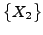of the previous static step (including large deformation stiffness and stress stiffness) and the stress stiffness matrix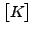of the buckling load .
• loop with starting value for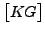• LU decomposition of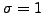• iterative calculation of the buckling factor with ARPACK
• determination of the buckling mode
• if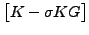buckling factor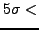exit loop, else set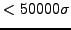buckling factor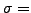and cycle
• determine the stresses and any other derived fields

The buckling mode in ARPACK (Mode 4, cf ) is used to solve a system of the form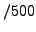(533)

whereis symmetric and positive definite andis symmetric but indefinite. The iterative procedure to find the eigenvalues requires routines to solve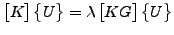(534)

and to calculate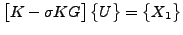(535)

Similar to the frequency calculations, the LU decomposition (SPOOLES) to solve Equation (534) is performed before the loop determining the buckling factor, since the left hand side of the equation does not vary. The matrix multiplication in Equation (535) is taken care of by routine op.f.

A major difference with the frequency calculations is that an additional iteration loop is necessary to guarantee that the value of the buckling factor is right. Indeed, experience has shown that the value of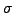matters here and that the inequalitybuckling factorshould be satisfied. If it is not, the whole procedure starting with the LU decomposition is repeated with a new value ofbuckling factor. If necessary, up to four such iterations are allowed.Next: Modal dynamic calculations Up: Filling and solving the Previous: Frequency calculations   Contents
guido dhondt 2018-12-15